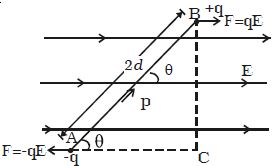Physics

# Equation for Electric Dipole in Uniform Electric Field

Electric Dipole in Uniform Electric Field:

Consider a dipole AB of dipole moment p placed at an angle θ in a uniform electric field E (Figure). The charge +q experience a force qE in the direction of the field. The charge –q experiences an equal force in the opposite direction. Thus the net force on the dipole is zero.The two equal and unlike parallel forces are not passing through the same point, resulting in a torque on the dipole, which tends to set the dipole in the direction of the electric field.

The magnitude of torque is,

τ = One of the forces x perpendicular distance between the forces

= F x 2d sin θ

= qE x 2d sin θ = pE sin θ (q * 2d = P)

In vector notation, τ = P * E